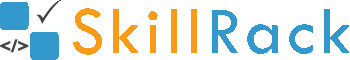ProgramID- 8090SkillRack
Odd Even Mixed

Accept two integers A and B as the input.
- If A and B are even then print "EVEN" as the output.
- If A and B are odd then print "ODD" as the output.
- If A is odd and B is even or if A is even and B is odd then print "MIXED" as the output.

Example Input/Output 1:
Input:
22 14

Output:
EVEN

Example Input/Output 2:
Input:
11 47

Output:
ODD

Example Input/Output 3:
Input:
34 97

Output:
MIXED

Max Execution Time Limit: 4000 millisecs